Math Olympiad Test: Triangles - 4

# Math Olympiad Test: Triangles - 4

Test Description

## 10 Questions MCQ Test Olympiad Preparation for Class 10 | Math Olympiad Test: Triangles - 4

Math Olympiad Test: Triangles - 4 for Class 10 2023 is part of Olympiad Preparation for Class 10 preparation. The Math Olympiad Test: Triangles - 4 questions and answers have been prepared according to the Class 10 exam syllabus.The Math Olympiad Test: Triangles - 4 MCQs are made for Class 10 2023 Exam. Find important definitions, questions, notes, meanings, examples, exercises, MCQs and online tests for Math Olympiad Test: Triangles - 4 below.
 1 Crore+ students have signed up on EduRev. Have you?
Math Olympiad Test: Triangles - 4 - Question 1

### Two poles of height 9 m and 14 m stand on a plane ground. If the distance between their feet is 12 m, then the distance between their tops is

Detailed Solution for Math Olympiad Test: Triangles - 4 - Question 1

Let AB and CD be the heights of given poles, BC be the distance between their feet and AD be the distance between their tops
Since, BCDE is a rectangle
∴ EB = DC = 9 m and ED = BC = 12 m
AE = AB – EB = 14 m – 9 m = 5m
Now, ΔAED is a right angled triangle
∴ AD2 = AE2 + ED2 [By Pythagoras theorem]
⇒ AD2 = (5)2 + (12)2 = 169 ⇒ AD = 13 m
Hence, the distance between their tops is 13 m.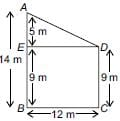Math Olympiad Test: Triangles - 4 - Question 2

### If a tree casts a 18 feet shadow and at the same time, a child of height 3 feet casts a 2 feet shadow, then the height of the tree is

Detailed Solution for Math Olympiad Test: Triangles - 4 - Question 2

Let AB be the height of tree which casts a shadow AC = 18 feet and ED be the height of child which casts a shadow CD = 2 feet
Now, in ΔABC and ΔDEC, we have
∠A = ∠D [Each 90°]
∠C = ∠C [Common angle]
∴ ΔABC ~ ΔDEC [By AA similarity]
⇒ AB/DE = AC/DC
⇒ AB/3 = 18/2
⇒ AB = 27 feet
So, the height of tree is 27 feet.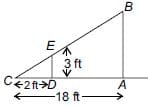Math Olympiad Test: Triangles - 4 - Question 3

### An aeroplane leaves an airport and flies due north at a speed of 1000 km per hour. At the same time, another aeroplane leaves the same airport and flies due west at a speed of 1200 km per hour. How far apart will be the two planes after 1(1/2) hours?

Detailed Solution for Math Olympiad Test: Triangles - 4 - Question 3

Let the point A represent the airport.
Let the plane-I fly towards North.
∴ Distance of the plane-I from the airport after 1(1/2) hours
= Speed × Time = 1000 × (1)1/2 km= 1500 km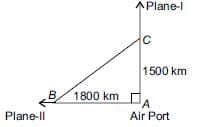Let the plane-II fly towards West.
∴ Distance of the plane-II from the airport after (1)1/2 hours
= 1200 × (1)1/2km = 1800 km
Now, in right ∆ABC, using Pythagoras theorem, we have
BC2 = AB2 + AC2
⇒ BC2 = (1800)2 + (1500)2 = 5490000
⇒ BC =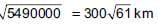Thus, after (1)1/2 hours, the two planes will be 300 √61 km apart from each other.

Math Olympiad Test: Triangles - 4 - Question 4

A 12 cm rod is held between a flashlight and a wall as shown. Find the length of the shadow on the wall if the rod is 45 cm from the wall and 15 cm from the light.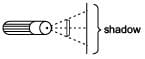Detailed Solution for Math Olympiad Test: Triangles - 4 - Question 4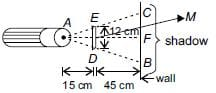∠DAM = ∠BAF (Common)
∠DMA = ∠BFM (Corresponding angles)
∴ ΔADM ~ ΔABF (By AA similarity)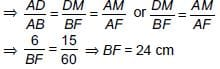∴ BC = BF + FC = 2BF = 48 cm (∵ CF = BF)

Math Olympiad Test: Triangles - 4 - Question 5

In the given figure, ABC is a right triangle right-angled at B. AD and CE are the two medians drawn from A and C respectively. If AC = 5 cm and AD =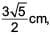then the length of CE is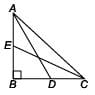Detailed Solution for Math Olympiad Test: Triangles - 4 - Question 5

Since ΔABD is a right angled triangle
∴ AD2 = AB2 + BD2 [By using Pythagoras theorem]
⇒ AD2 = AB2 + (BC/2)2 [∵ AD is median on BC]
⇒ AD2 = AB2 + (1/4) BC2 ...(i)
Similarly in, ΔBCE
CE2 = BC2 + BE2 ⇒ CE2 = BC2 + (AB/2)[∵ EC is median on AB]
⇒ CE2 = BC2 + (1/4).AB2 ...(ii)
Adding (i) and (ii), we get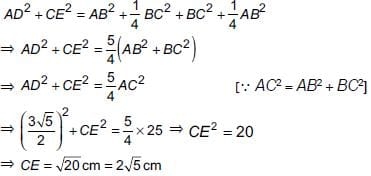Math Olympiad Test: Triangles - 4 - Question 6

Mason Construction wants to connect two parks on opposite sides of town with a road. Surveyors have laid out a map as shown. The road can be built through the town or around town through point R. The roads intersect at a right angle at point R. The line joining Park A to Park B is parallel to the line joining C and D.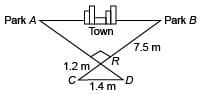(i) What is the distance between the parks through town?
(ii) What is the distance from Park A to Park B through point R?

Detailed Solution for Math Olympiad Test: Triangles - 4 - Question 6

(i) Since, CD || AB [Given]
∴ In ΔRCD and ΔRBA, we have
∠BAR = ∠RDC [Alternate interior angles]
∠ABR = ∠RCD
∴ ΔRCD ~ ΔRBA [By AA similarity]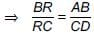... (i)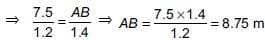i.e., Distance between the parks through town is 8.75 m.
(ii) In right ΔCRD, we have
(CD)2 = (CR)2 + (RD)2
⇒ RD2 = (1.4)2 – (1.2)2 = 0.52 ⇒ RD = 0.72 m
Since ΔRCD ~ ΔRBA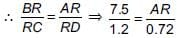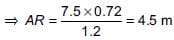i.e., Distance from Park A to Park B through point R = AR + RB
= 4.5 m + 7.5 m = 12 m

Math Olympiad Test: Triangles - 4 - Question 7

Which of the following statements is CORRECT?

Detailed Solution for Math Olympiad Test: Triangles - 4 - Question 7

BPT (Basic proportional theorem) states that if a line is drawn parallel to one side of a triangle to intersect the other two sides in distinct points then the other two sides are divided in the present ratio.

Math Olympiad Test: Triangles - 4 - Question 8

Match the following.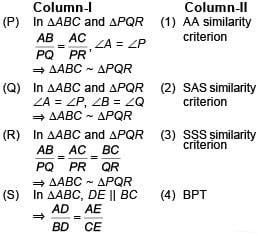Detailed Solution for Math Olympiad Test: Triangles - 4 - Question 8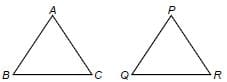(P) Given :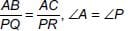∴ ∠A is containing the sides AB and AC and ∠P is containing the sides PQ and PR.
∴ ΔABC ~ ΔPQR (By SAS criteria)
(Q) Given : ∠A = ∠P, ∠B = ∠Q
∴ ΔABC ~ ΔPQR (By AA criteria)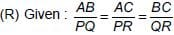∵ Sides of the ΔABC and ΔPQR are in proportion
∴ ΔABC ~ ΔPQR (By SSS criteria)
(S) Given, DE || BC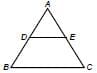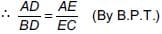Math Olympiad Test: Triangles - 4 - Question 9

In the given figure,the line segment XY is parallel to side AC of ΔABC and it divides the triangle into two parts of equal area. Then, find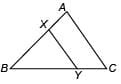(i) AX : AB (ii) AC/XY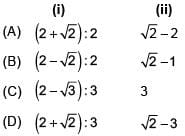Detailed Solution for Math Olympiad Test: Triangles - 4 - Question 9

(i) Since, XY || AC, we have
∠A = ∠BXY and ∠C = ∠BYX [Corresponding angles]
∴ ΔABC ~ ΔXBY [By AA similarity]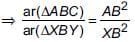...(i)
But, ar(ΔABC) = 2 × ar(ΔXBY) [Given]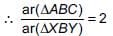...(ii)
From (i) and (ii), we get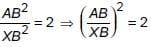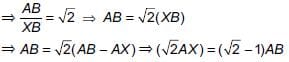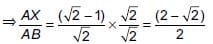Hence, AX : AB = (2 − √2) : 2
(ii) Since, ΔABC ~ ΔXBY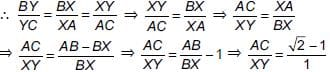Math Olympiad Test: Triangles - 4 - Question 10

P and Q are the mid-points of the sides CA and CB respectively of a DABC, right angled at C, then find:
(i) 4AC2 + BC2
(ii) 4BC2 + AC2
(iii) 4(AQ2 + BP2)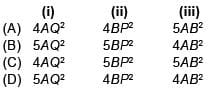Detailed Solution for Math Olympiad Test: Triangles - 4 - Question 10

Construction: Join PQ, BP and AQ.
(i) 4AC2 + BC2 = 4AC2 + (2QC)2
[∵ Q is mid-point of BC]
= 4AC2 + 4QC2
= 4(AC2 + QC2)
= 4AQ2 [∵ AQC is right angled triangle]
(ii) 4BC2 + AC2 = 4BC2 + (2CP)2 [∵ P is mid-point of AC]
= 4BC2 + 4CP2 = 4(BC2 + CP2) = 4BP2 [∵ PBC is right angled triangle]
(iii) 4[AC2 + QC2] = 4(AQ)2 [from (i) part]
⇒ 4AC2 + BC2 = 4AQ2 ...(i)
4[BC2 + CP2] = 4(BP)2 [from (ii) part]
⇒ 4BC2 + AC2 = 4BP2 ...(ii)
Now, adding (i) and (ii), we get
4(AQ2 + BP2) = 5(AC2 + BC2) = 5AB2 [∵ ABC is right angled triangle]

## Olympiad Preparation for Class 10

11 videos|36 docs|201 tests
 Use Code STAYHOME200 and get INR 200 additional OFF Use Coupon Code